We notice that you are using AdBlock. Please whitelist Mortgage Calculator to help keep this site up and running! :)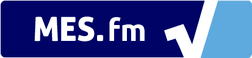# Mortgage Calculator Tutorial

Using our mortgage calculator is very simple. Just fill in the calculator inputs and all the calculations are done automatically. The dropdown buttons can be shown or hidden depending on the data that you would like to see.

In this article (as well as in the above embedded video), I will go over an example and breakdown each of the calculations as well as the formulas behind them.

## Example of a Mortgage Repayment Plan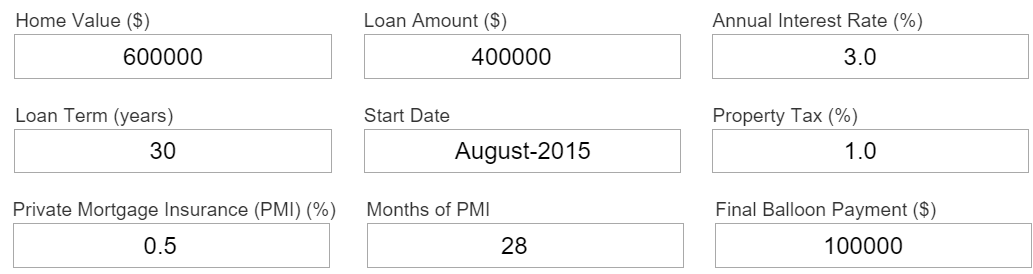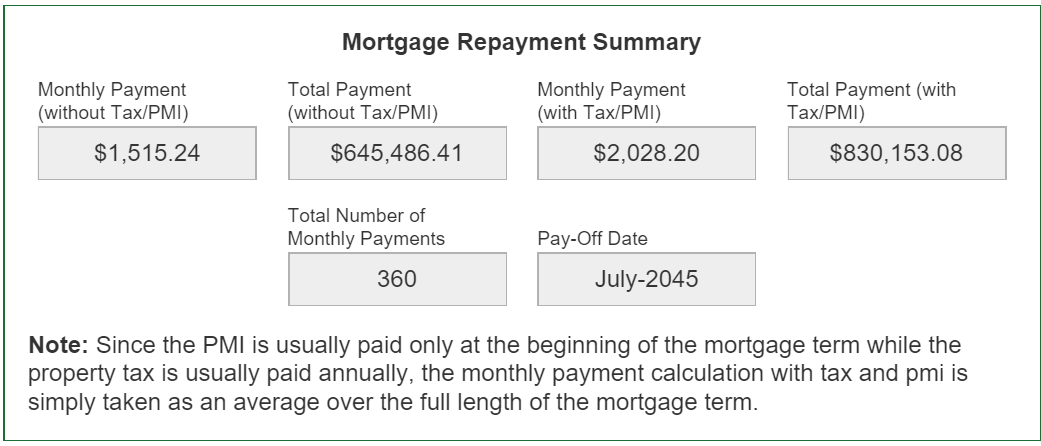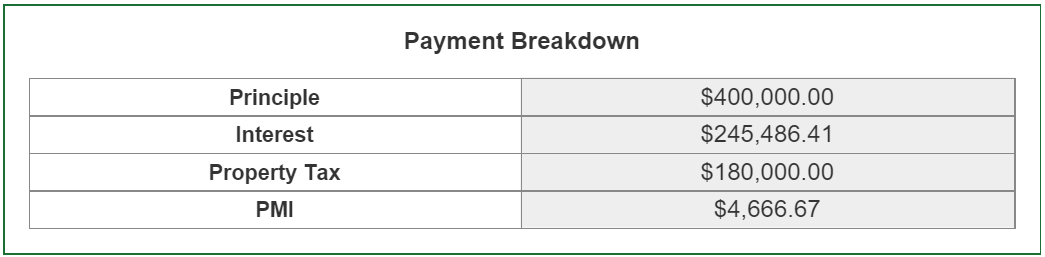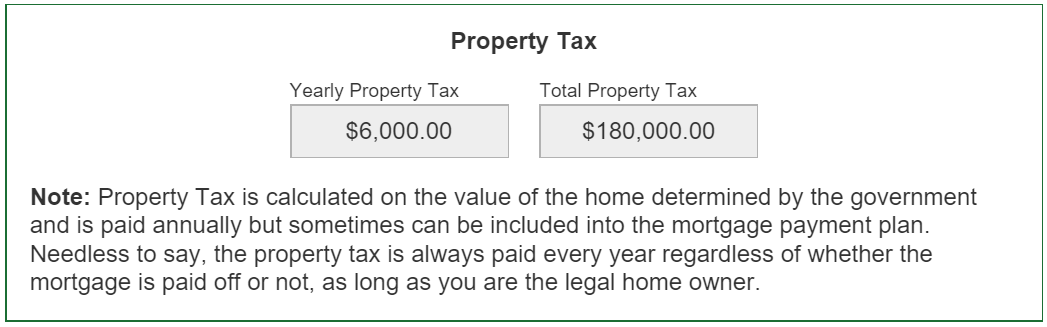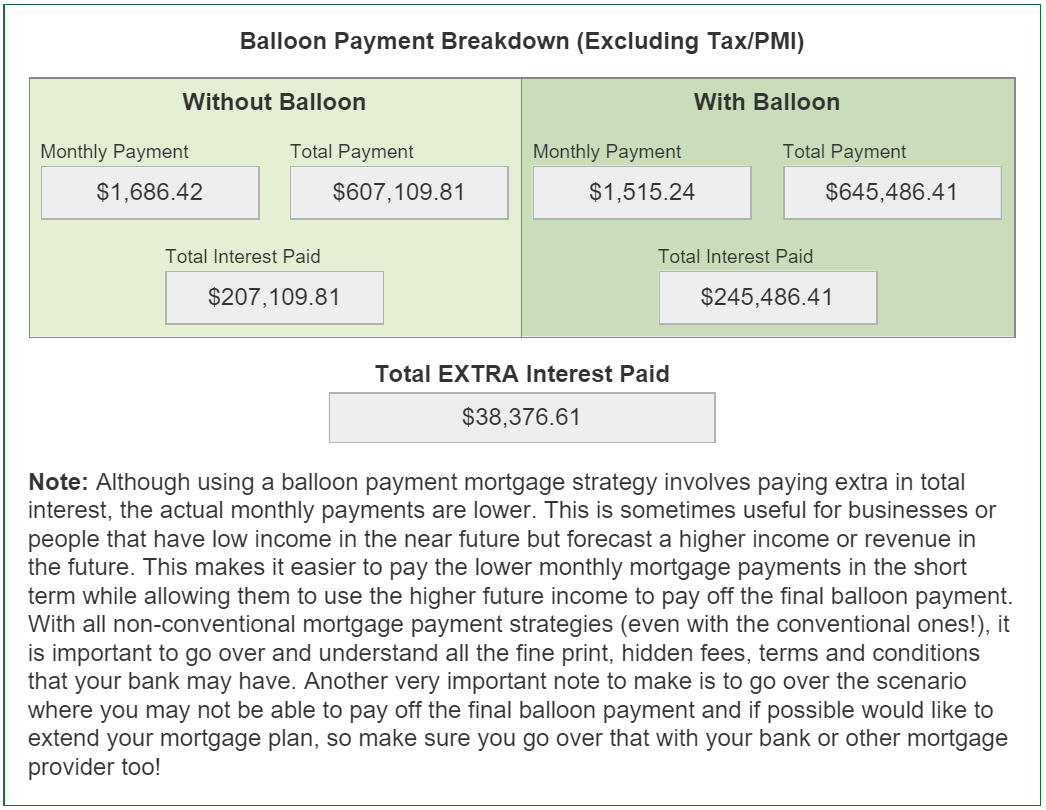## Breakdown of the Calculations in our Mortgage Calculator

### Monthly Payment (without Tax/PMI) Calculation

The first calculation that the mortgage calculator solves is the Monthly Payment (without Tax/PMI), which in our example is $1,515.24. This calculation is from the Mortgage Amortization Formula (including Balloon Payment) and is shown below: A = \frac{iP(1+i)^n}{(1+i)^n - 1} - \frac{iB}{(1+i)^{n+1} - (1+i)} where: • A = periodic payment amount • P = amount of principle or loan owing (subtracting any down-payments) • i = period interest rate • n = total number of payments • B = Final Balloon Payment • If B = 0, then the amortization formula is the same as the basic one In our example, the interest rate given is 3% and is the annual interest rate or sometimes referred to the annual percentage rate (APR). But since we are dealing with monthly payments we need to obtain the monthly interest and this can be simply found by dividing the APR by 12 months: i_m = \frac{i_m}{12} = \frac{3\%}{12} = \frac{.03}{12} = 0.0025 The rest of variables in the above formula, for our given example, are shown below: 1 + i_m = 1.0025 n = 12 ~ years * 12 \frac{months}{year} = 360 ~ months P = \$400,000
B = \$100,000 Now inputting these variables into the amortization formula we obtain: A_0 = Monthly Payment (without Tax/PMI) = \frac{0.0025 * 400,000 * 1.0025^{360}}{1.0025^{360} - 1} - \frac{0.0025 * 100,000}{1.0025^{361} - 1.0025} = 1686.42 + 171.18 = \$1515.24

### Total Payment (without Tax/PMI) Calculation

The Total Payment (without Tax/PMI) is simply equal to the sum of the monthly payments plus the final balloon payment:

T_0 = Total ~ Payment ~ (without Tax/PMI)
= A_0 * 360 + B(1 + \frac{1}{1 + i})
= 1515.24 * 360 + 100,000
= 545,486.41 + 100,000
= \$645,486.41 ### Payment Breakdown: Interest, Property Tax and PMI The total interest paid can be calculated by subtracting the loan amount from the actual Total Payment (without Tax/PMI) and is calculated below: T_i = Total ~ Interest ~ Paid = T_0 - P = 645,486.41 - 400,000 = \$245,486.41

The property tax is calculated on a per year basis and is simply the estimated Home Value multiplied by property tax rate:

T_p = 600,000 ~ home ~ value * 1\% ~ tax * 30 ~ years
= 600,000 * 0.01 * 30 = \$180,000 The Private Mortgage Insurance (PMI) is calculated by multiplying the total mortgage loan amount by the PMI rate (averaged out to a monthly percentage rate) and multiplied by the length of the insurance term for the total amount: T_{PMI} = 400,000 ~ mortgage ~ loan * \frac{0.5\% ~ PMI}{12 ~ months} * 28 ~ months = 400,000 * \frac{0.005}{12}*28 = \$166.67 * 28 = \$4666.67 ### Monthly and Total Payment with Tax/PMI Including the property tax and PMI into the mortgage payments is a tricky situation because property tax is usually paid in a per year basis, PMI is usually paid for a specific number of months in the short term, while mortgage payments are usually monthly across a long period. To simplify the calculations process, in our Mortgage Calculator the Payments with Tax/PMI are included by simply averaging out the Total Property Tax and PMI over a per month basis across life of the mortgage. A = Monthly ~ Payment ~ (with ~ Tax/PMI) = A_0 + \frac{T_p + T_{PMI}}{360 ~ months} = 1515.24 + \frac{4666.67 + 180000}{360} = 1515.24 + 512.96 = \$2028.20
T = Total ~ Payment ~ (with ~ Tax/PMI)
= A * 360 ~ months + B(1 + \frac{i}{1 + i})
= 2028.60 * 360 + 100,000 = \$830,153.08 ### Bi-Weekly Payment Strategy (Excluding Tax/PMI) The Bi-Weekly payment strategy is a way of saving on interest by paying more frequently. The payments are paid every 2 weeks but the bi-weekly payments are usually just half the monthly payments, as in our Mortgage Calculator. A_w = \frac{A_0}{2} = \$757.62

Now the important part to remember with a bi-weekly payment strategy is that the length of the mortgage amortization term is actually less than that for a monthly payment strategy because of the more frequent payments which lower the total amount interest gets compounded. Thus in order to calculate the Total Bi-Weekly payment we need to solve for the length of the mortgage term. We can do this by rearranging the mortgage amortization formula to solve for n:

A = P\frac{i(1 + i)^n}{(1+i)^n - 1} - \frac{iB}{(1+i)^{n+1} - (1 + i)}
A = P\frac{i(1 + i)^n}{(1+i)^n - 1} - \frac{iB}{(1+i)((1+i)^n - 1)}
A(1+i)^n - A = iP(1+i)^n - \frac{iB}{1+i}
(1+i)^n(A - iP) = A - \frac{iB}{1+i}
(1+i)^n = \frac{A - \frac{iB}{1+i}}{A - iP}
nln(1+i) = ln|\frac{A - \frac{iB}{1+i}}{A - iP}|
n = \frac{ln|\frac{A - \frac{iB}{1+i}}{A - iP}|}{ln|1+i|}

Now that the formula for n number of payments periods is derived, we can start inputting the variables while noting making sure to account for the bi-weekly interest rate which is the APR divided by the 26 bi-weeks in a year.

i_w = \frac{i_a}{26 ~ bi-weeks} = \frac{3}{100*26} = \frac{3}{2600}
1+i_w = \frac{2600}{2600} + \frac{3}{2600} = \frac{2603}{2600}
n_w = number ~ of ~ bi-weeks
= ln|\frac{757.62 - \frac{3}{2600} * \frac{100,000}{\frac{2603}{2600}}}{756.62 - \frac{3}{2600} * 400,000}| ~ \div ~ {ln|\frac{2603}{2600}|}
\approx 671.64 ~ bi-weeks \approx 672 ~ bi-weeks

Now the Total Payments will be calculated similarly to that for the Mortgage repayment strategy as well as account for the balloon payment (plus interest):

T_w = A_w * n_w + B(1 + \frac{i}{1 + i})
= 757.62 * 671.64 + 100,000
= \$608,850.88 The total interest paid and saved using the bi-weekly payment strategy as compared to the monthly payment strategy is calculated below: T_{iw} = Total ~ Bi-Weekly ~ Interest = T_w - P = 608,850.88 - 400,000 = \$208,850.88
Total ~ Interest ~ Saved = T_i = T_{iw}
= 245,486.41 - 208,850.88 = \$36,635.53 ### Balloon Payment Breakdown (Excluding Tax/PMI) The last calculation to evaluate is the extra interest paid when using the balloon payment strategy. Thus we need to first calculate the monthly and total payments without the balloon payment and compare to with the balloon payment. The monthly payment is simply the first part of the mortgage amortization formula and is calculated below: A_{0B} = Monthly ~ Payment ~ (no ~ Tax/PMI/Balloon) = \frac{0.0025 * 400,000 * 1.0025^{360}}{1.0025^{360} - 1} =$1686.42
T_{0B} = A_{0B} * 360 ~ months
= 1686.42 * 360 = \$607,109.81 T_{iB} = T_{0B} - P = 607,109.81 - 400,000 = \$207,109.81

As predicted the total interest without the balloon payment and this is because the total monthly payments are higher.. The total extra interest paid using the balloon payment strategy is calculated below:

Total ~ Extra ~ Interest ~ Paid = T_i - T_{iB}
= 245,486.41 - 207,109.81
= \\$38,376.61

Although the total extra interest paid is substantial, it does lower the monthly payments and many times businesses use the balloon strategy because of lower initial revenue streams.

If you have any questions about our Mortgage Calculator, the calculations I went over, or any suggestions on making it even better please let us know by commenting below! :)